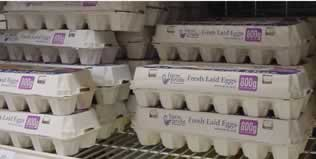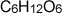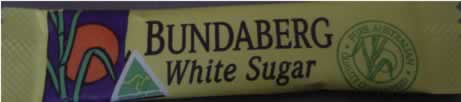Mole to mass via the formula mass Remember the comparison of the dozen and the mole? We will revisit this for a moment.What is the mass of these 23 dozen eggs? Before we can answer this question we need to know the mass of one dozen eggs. If the mass of one dozen eggs is 1kg then surely the mass of the entire section is 1kg X 23. The sugar packet on the left contains 2.5 mole of sugar. The chemical formula for sugar is. We can work out its mass. The formula mass tells us the mass, in grams, of one mole of sugar. If we know there are 2.5 mole of sugar in the packet we can work out the mass by multiplying the formula mass(190 atomic mass units) by the number of mole. So the mass of sugar is 190 X 2.5=475gramsHome